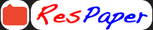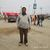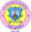Trending ▼   ResFinder# ICSE Class X Prelims 2019 : Physics (St. Peters School, RaeBareli)

3 pages, 61 questions, 47 questions with responses, 68 total responses,20Pawan Agnihotri St. Peter's School, RaeBareli
+Fave Message
 Home > stpeters1983 >   F Also featured on: School PageFormatting page ...

Half yearly Examination: 2018 Class: 10 SCIENCE Paper 1(PHYSICS) Time: 2 hrs. Max.marks: 80 You will not be allowed to write during the first 15 minutes. This time is to be spent in reading the question paper. The time given at the head of this paper is the time allowed for writing the answer. Section 1 is compulsory. Attempt any four questions from section 2. The intended marks for questions or parts of question are given in ( ) Section 1 Question 1: [5 Questions 2=10] a) Name the force required for uniform circular motion .State its direction. b) State factors on which the position of centre of gravity of a body depend. Explain with example. c) A body is acted upon by a force .State two conditions when the work is zero. d) A machine works as a (1) force multiplier (2) speed multiplier. In each case whether the velocity ratio is more than or less than 1. e) For which colour of white light, is the refractive index of a transparent medium (1) the least (2) the most? Question 2: [5 Questions 2=10] -2 a) How fast a man weighing 600N run so that his kinetic energy is 750 J .( g = 10ms ) b) A force of 5 kgf is required to cut a metal sheet .A shears used for cutting the metal sheet has its blades 5 cm long, while its handles are 10 cm long. What effort is needed to cut the metal; sheet? c) The refractive index of water is 4/3 and of glass is 3/2.What is the refractive index of glass with respect to water? d) Express the power (with sign) of a concave lens of focal length 20 cm. e) The wavelength of X rays is 0.01 A. Calculate its frequency. State the assumption made, if any. Question 3: [5 Questions 2=10] a) Explain the term critical angle with the aid of labelled diagram. b) What is lateral displacement? Draw a ray diagram showing the lateral displacement of a ray of light when it passes through a parallel sided glass slab. c) State the condition for each of the following; I. A lens has both its focal lengths equal II. A ray passes undeviated through the lens. d) A concave lens forms the image of an object kept at a distance of 20 cm in front of it,at a distance 10 cm on the side of the object.(1) What is the nature of the image? (2)Find the focal length of lens? e) Draw a neat labelled ray diagram to show the formation of image by a magnifying glass. State three characteristics of the image. Question 4: [5 Questions 2=10] a) What is the range of wavelength of the spectrum of white light in (1) A (2) nm? b) Name one electromagnetic wave of wavelength smaller than that of violet light. State its one use. c) What should be the minimum distance between the source and reflector in water so that the echo is heard distinctly? The speed of sound in water is 1400 ms-1. d) Name the unit used to measure the sound level. e) State the factor that determines pitch of a note and the loudness of the sound heard. Section 2 Question 5: [4+3+3=10] a) The wheel shown in the diagram has a fixed axle passing through O The wheel is kept stationary under the action of (i) a horizontal force F1 at A and (ii) a vertical force F2 at B. 1) Show the direction of force F2 in the diagram. 2) Which of the force F1 or F2 is greater? 3) Find the ratio between the forces F1 and F2.Given: AO = 2.5cm, BO = 1.5 cm and O O = 2 cm.Formatting page ...

Top Contributors
to this ResPaperDeadpool(14)Krish Rajiv Nair(13)Aditya Dungrani(10)Megan Sequeira(8)Formatting page ...Tags : ISC Physics Half yearly question paper 2018